# CV深度学习面试问题记录

Posted by kevin on March 10, 2020

## RPN是怎么做的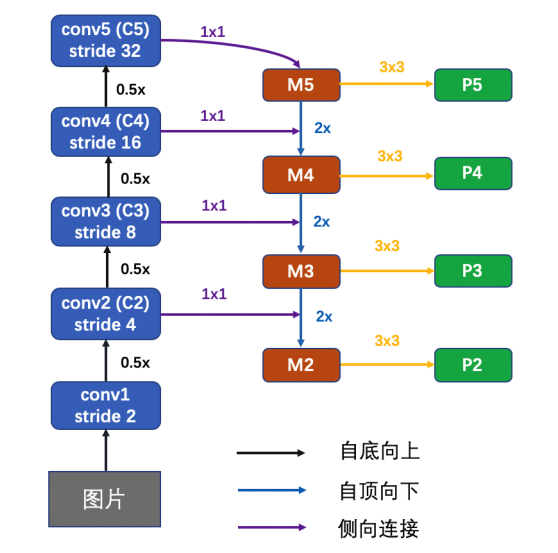## 过拟合欠拟合是啥，怎么解决？

### 过拟合

1. 增加更多的训练集或者数据增强(根本上解决问题)
2. 采用更简单点的模型(奥卡姆剃刀准则，简单的就是正确的)
3. 正则化(L1，L2，Dropout)
4. 可以减少不必要的特征或使用较少的特征组合

## ResNet为啥能够解决梯度消失？怎么做的，能推导吗？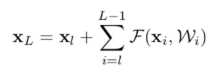• 输入是x；
• F(x)相当于residual，它只是普通神经网络的正向传播；
• 输出是这两部分的加和H(x) = F(x)（就是residual） + x（就是shortcut，此代码shortcut部分做了一次卷积，也可以不做）；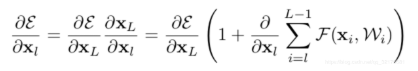• 对x的直接映射，梯度为1；
• 通过多层普通神经网络映射结果为：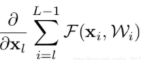cxyzjd.com/article/qq_32172681/100177636

## 深度学习里面PCA的作用

PCA 用于降低维度，消除数据的冗余，减少一些不必要的特征。常用步骤如下（设有 m 条 n 维数据）：

1. 将原始数据按照列组成 m 行 n 列矩阵 X
2. 将 X 的每一列(代表一个属性字段)进行零均值化(去中心化)减去这一列的均值得到特征中心化后的矩阵 $B = X_i - \mu_i$
3. 求出协方差矩阵 $C = (X_i - \mu_i)*(X_i - \mu_i)^T$
4.  求出矩阵 C 的特征值($det A-\lambda I =0$)和特征向量，按照特征值的大小将对应的特征向量从左往右排列，取前 k 个最大的特征值对应的特征向量组成矩阵 P
5. Y = PX 即为降维到 k 维后的数据，即从 n 维降到了 k 维，保留了原本的大部分信息

reference:

https://blog.csdn.net/Virtual_Func/article/details/51273985

## YOLOv3的框是怎么聚出来的？

YOLOv3 的框是作者通过对自己的训练集的 bbox 聚类聚出来的，本质上就是一个 kmeans 算法，原理如下：

1. 随机选取 k 个类别的中心点，代表各个聚类
2. 计算所有样本到这几个中心点的距离，将每个样本归为距离最小的类别
3. 更新每个类别的中心点(计算均值)
4. 重新进行步骤 2 ，直到类的中心点不再改变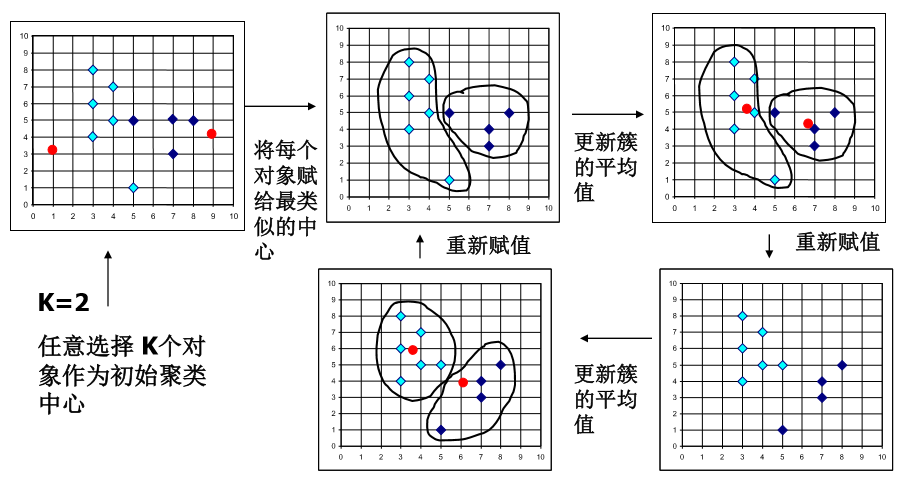YOLOv3 的 kmeans 想法不能直接用 w 和 h 来作为聚类计算距离的标准，因为 bbox 有大有小，不应该让 bbox 的尺寸影响聚类结果，因此，YOLOv3 的聚类使用了 IOU 思想来作为距离的度量 $$d(bbox, centroid) = 1 - IOU(bbox, centroid)$$ 也就是说 IOU 越大的话，表示 bbox 和 box_cluster 就越类似，于是将 bbox 划归为 box_cluster

import numpy as np
def kmeans(boxes, k, dist=np.median):
"""
Calculates k-means clustering with the Intersection over Union (IoU) metric.
:param boxes: numpy array of shape (r, 2), where r is the number of rows
:param k: number of clusters
:param dist: distance function
:return: numpy array of shape (k, 2)
"""
rows = boxes.shape

distances = np.empty((rows, k))
last_clusters = np.zeros((rows,))

np.random.seed()

# the Forgy method will fail if the whole array contains the same rows
# 随机 sample k 个 box 作为聚类中心
clusters = boxes[np.random.choice(rows, k, replace=False)]

while True:
for row in range(rows):
# 计算每一个 box 到聚类中心的距离（用 IOU 衡量距离）
distances[row] = 1 - iou(boxes[row], clusters)
# 选一个最近的聚类中心
nearest_clusters = np.argmin(distances, axis=1)
# 聚类中心不变的话就结束聚类
if (last_clusters == nearest_clusters).all():
break

for cluster in range(k):
# 根据聚类结果更新聚类中心
clusters[cluster] = np.median(boxes[nearest_clusters == cluster], axis=0)

last_clusters = nearest_clusters

return clusters



reference:

https://github.com/AlexeyAB/darknet/blob/master/scripts/gen_anchors.py

https://www.cnblogs.com/sdu20112013/p/10937717.html

## FCOS 存在什么问题，如何解决

1. 分配正负样本时，只要 anchor 在 gt_box 里面就算是正样本，这样会将很多背景区域也当成正样本，解决方式就是用 center_sampling，只在 gt_box 周围一个范围内的样本才是正样本。
2. 原始论文中，为了解决网络在不同 FPN 层之间回归的距离差异太大，假设 FPN 某个 stride feature map 上某个点的回归预测值为 x，则对应的值为 $\exp^{S_i*x}$​，使输出非负数，其中 $S_i$​​ 为当前尺度的一个可学习参数。这样做的话，用到指数函数，并且效果也不一定好，改进的点就是先将预测的距离通过一个 ReLU 使结果非负，然后在做标签的时候就将距离除以 stride，这样子的话每一层要回归的距离范围就差不多了，推理的时候只需要乘上 stride 就能还原真实 box 了。
3. centerness 没什么作用，直接预测 IoU 代替。
4. bbox loss weight 在原文中所有正样本的权重都是一样的，改进的话可以将该点的 centerness 值作为权重，越靠近中心点的 box 给越大的权重。

FCOS 的改进 trick - 极市社区 (cvmart.net)

## 说一下RCNN发展史

RCNN -> Fast RCNN -> Faster RCNN

#TODO

### Faster RCNN

training 阶段，RPN 网络提出了 2000 左右的 proposals，这些 proposals 被送入到 Fast R-CNN 结构中，在 Fast R-CNN 结构中，首先计算每个 proposal 和 gt 之间的 iou，通过人为的设定一个 IoU 阈值（通常为 0.5），把这些 Proposals 分为正样本（前景）和负样本（背景），并对这些正负样本采样，使得他们之间的比例尽量满足（1:3，二者总数量通常为 128），之后这些 proposals（128 个）被送入到 Roi Pooling，最后进行类别分类和 box 回归。inference 阶段，RPN 网络提出了 300 左右的 proposals，这些 proposals 被送入到 Fast R-CNN 结构中，和 training 阶段不同的是，inference 阶段没有办法对这些 proposals 采样（inference 阶段肯定不知道 gt 的，也就没法计算 iou），所以他们直接进入 Roi Pooling，之后进行类别分类和 box 回归

## 各种边缘检测算法的原理 (Sobel, Canny, Laplacian)

### Sobel 算子

Sobel 算子求的是一阶导数，后面有些边缘检测算子求的是二阶导数，并且 Sobel 在使用 3*3 卷积的时候效果没有 Scharr 滤波器更好，所以如果用 3*3 卷积核进行滤波的话尽量使用 Scharr 滤波器（卷积核的数值比 Sobel 更大）。

$G_{x} = \begin{bmatrix} -1 & 0 & +1 \\ -2 & 0 & +2 \\ -1 & 0 & +1 \end{bmatrix} * I \\\\ G_{y} = \begin{bmatrix} -1 & -2 & -1 \\ 0 & 0 & 0 \\ +1 & +2 & +1 \end{bmatrix} * I \\\\ G = \sqrt{ G_{x}^{2} + G_{y}^{2} } \ or \ G = |G_{x}| + |G_{y}|$

### Laplacian 算子

laplacian 算子在 Sobel 的基础上进行改进，Sobel 那会儿已知图像的边缘部分是邻域梯度最大的部分，那么再对这个求一次导数呢，那么边缘部分的二阶导数就是 0 了。真不错，因此 laplacian 算子的原理就是对图像求二阶导数，不过预处理部分和 Sobel 差不多，也是要先转化成灰度图，再高斯滤波去除噪声，再进行求导操作。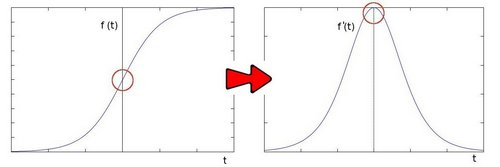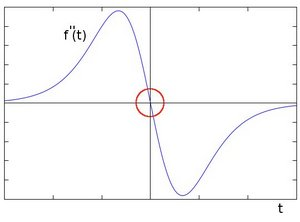laplacian 算子运用的卷积核是如下数值的，因为还是求导数，所以内部调用的是 Sobel 函数。 $$\begin{bmatrix} 0 & +1 & 0 \\ 1 & -4 & 1 \\ 0 & +1 & 0 \end{bmatrix}$$

### Canny 算子

Canny 算子是一种性能更好的算子，原理分为几步：

1. 预处理（高斯去噪，灰度化）

2. 对图像进行差分求导（Sobel）得到梯度方向和角度

3. 进行极大值抑制去除真正的边缘周围的杂质得到比较好的边缘

1. 这一步的目的是将模糊（blurred）的边界变得清晰（sharp）。通俗的讲，就是保留了每个像素点上梯度强度的极大值，而删掉其他的值。对于每个像素点，进行如下操作：     a) 将其梯度方向近似为以下值中的一个（0,45,90,135,180,225,270,315）（即上下左右和 45 度方向）

b) 比较该像素点，和其梯度方向正负方向的像素点的梯度强度

c) 如果该像素点梯度强度最大则保留，否则抑制（删除，即置为 0）

4. 双阈值筛选更精细的边缘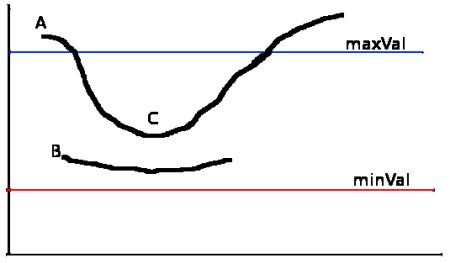## 关于相机标定以及姿态估计

### 相机内外参是什么意思### pnp 问题怎么解

https://docs.opencv.org/3.4/d5/d1f/calib3d_solvePnP.html

https://zhuanlan.zhihu.com/p/389653208

## 边框回归怎么做的，为什么需要 Encode

Most recently object detection programs have the concept of anchor boxes, also called prior boxes, which are pre-defined fix-sized bounding boxes on image input or feature map. The bounding box regressor, instead of predicting the bounding box location on the image, predicts the offset of the ground-truth/predicted bounding box to the anchor box. For example, if the anchor box representation is [0.2, 0.5, 0.1, 0.2], and the representation of the ground-truth box corresponding to the anchor box is [0.25, 0.55, 0.08, 0.25], the prediction target, which is the offset, should be [0.05, 0.05, -0.02, 0.05]. The object detection bounding box regressor is trying to learn how to predict this offset. If you have the prediction and the corresponding anchor box representation, you could easily calculate back to predicted bounding box representation. This step is also called decoding.

https://leimao.github.io/blog/Bounding-Box-Encoding-Decoding/

https://blog.csdn.net/zijin0802034/article/details/77685438

https://www.zhihu.com/question/370354869

gt 在做 loss 之前就已经和 Anchor 经过了 encode ，也就是从 Anchor 到 gt 所要经过的线性变换 (Gx, Gy, Gw, Gh)，回归网络的输出就是这四个变换，也就是说网络在学习这种映射关系。所以网络的输出并不是真正的坐标，得到坐标只需要根据 encode 得到 decode 函数做一下解码就行了。

## 常见的Loss，回归的，分类的，Focal Loss

### Sigmoid

$\sigma=\frac{1}{1+e^-x}$

### Focal Loss

def FocalLoss(self, logit, target, gamma=2, alpha=0.5):
n, c, h, w = logit.size()
criterion = nn.CrossEntropyLoss(weight=self.weight, ignore_index=self.ignore_index,
size_average=self.size_average)
if self.cuda:
criterion = criterion.cuda()

logpt = -criterion(logit, target.long())
pt = torch.exp(logpt)
if alpha is not None:
logpt *= alpha
loss = -((1 - pt) ** gamma) * logpt

if self.batch_average:
loss /= n

return loss


reference: https://www.cnblogs.com/king-lps/p/9497836.html

FocalLoss 对样本不平衡的权重调节和减低损失值 - 知乎 (zhihu.com) 这个和 CE BCE 进行对比，比较清晰，但是这两个都是基于 softmax 的 FocalLoss，mmdet 中默认是基于 sigmoid 的 BCE loss 作为原型进行 FocalLoss 的。

### softmax

$\sigma(Z)_j = \frac{e^{z_j}}{\sum^K_{k=1}e{z_k}}$
import numpy as np
z = np.array([1.0, 2.0, 3.0, 4.0, 1.0, 2.0, 3.0])
print(np.exp(z)/sum(np.exp(z)))
>>> [0.02364054 0.06426166 0.1746813 0.474833 0.02364054 0.06426166 0.1746813 ]


### BCELoss

$L = -\frac{1}{n}(ylog(y_{pred})+(1-y)log(1-y_{pred}))$

### CrossEntropyLoss

$CE = -\frac{1}{n}ylog(y_{pred})$

$CE = -\frac{1}{n}ylog(\frac{e^{y_c}}{\sum_je^{y_j}})$

>>> i = torch.randn(3,3)
>>> i
tensor([[-1.8954, -0.3460, -0.6694],
[ 0.5421, -1.1799,  0.8239],
[-0.0611, -0.8800,  0.8310]])
>>> label = torch.tensor([[0,0,1],[0,1,0],[0,0,1]])
>>> label
tensor([[0, 0, 1],
[0, 1, 0],
[0, 0, 1]])
>>> sm = nn.Softmax(dim=1)
>>> sm(i)
tensor([[0.1097, 0.5165, 0.3738],
[0.3993, 0.0714, 0.5293],
[0.2577, 0.1136, 0.6287]])
>>> torch.log(sm(i))
tensor([[-2.2101, -0.6607, -0.9840],
[-0.9180, -2.6400, -0.6362],
[-1.3561, -2.1751, -0.4640]])
>>> ll = nn.NLLLoss()
>>> target = torch.tensor([2,1,2])
>>> ll(torch.log(sm(i)), target)
tensor(1.3627)

>>> i
tensor([[-1.8954, -0.3460, -0.6694],
[ 0.5421, -1.1799,  0.8239],
[-0.0611, -0.8800,  0.8310]])
>>> los = nn.CrossEntropyLoss()
>>> los(i, target)
tensor(1.3627)


## L1、L2范数是什么，区别呢？

reference:

https://zhuanlan.zhihu.com/p/28023308

https://www.cnblogs.com/LXP-Never/p/10918704.html（解释得挺好的，借鉴了下面这篇文章）

http://www.chioka.in/differences-between-l1-and-l2-as-loss-function-and-regularization/#

https://wangjie-users.github.io/2018/11/28/%E6%B7%B1%E5%85%A5%E7%90%86%E8%A7%A3L1%E3%80%81L2%E8%8C%83%E6%95%B0/

## pooling layer怎么反向传播

• average pooling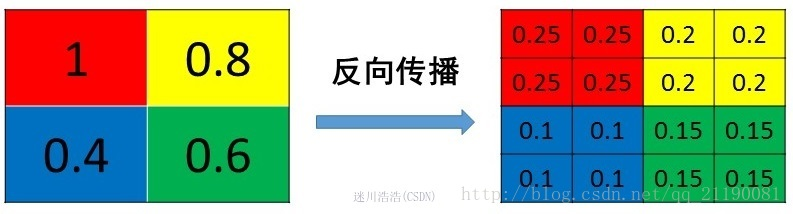• max pooling

max pooling要记录下最大值像素的index，然后在反向传播时将梯度传递给值最大的一个像素，其他像素不接受梯度，也就是为0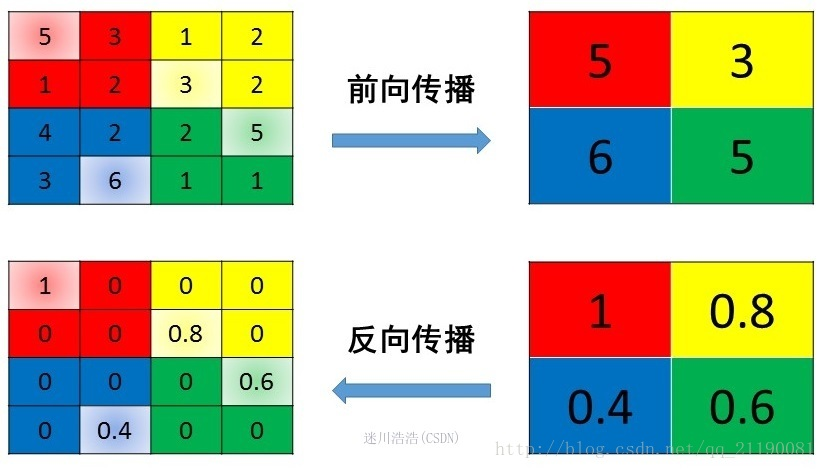reference: https://blog.csdn.net/qq_21190081/article/details/72871704

## MobileNet在特征提取上有什么不同

MobileNet 用的是深度可分离卷积（Depthwise separable convolution），将传统的卷积分成了 Depthwise convolution 和 Pointwise convolution，也就是先对每个通道进行单独的卷积，不把这些特征组合在一起，然后用 Pointwise convolution 也就是一个 1* 1 卷积核将通道特征组合在一起，这样可以大大减少模型的参数量以及运算量，适合运用于嵌入式设备等对延时和性能要求较高的场景中。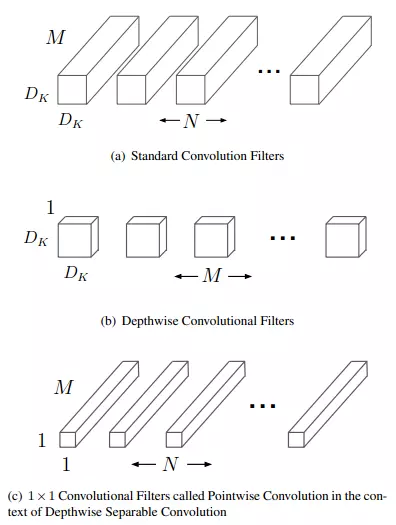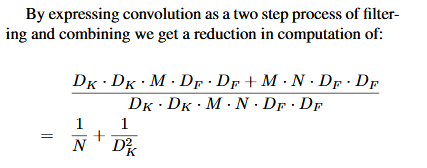Depthwise convolution 的实现是通过 nn.Conv2d 中的 groups 参数，这个数字要能被 in_channels 和 out_channels 整除，一般来说，Depthwise convolution 中的第一个 Group convolution 的输入输出通道都是一样的，pointwise convolution 才是起到改变维度的作用。下面例子中通过通道可分离卷积保存的模型比标准卷积更小 (47KB VS 362KB)

import torch.nn as nn

class Normal(nn.Module):
def __init__(self, i, o, k):
super(Normal, self).__init__()
self.conv = nn.Conv2d(i, o, k, padding=k // 2)
def forward(self, x):
x = self.conv(x)
return x

class DepwiseSeparable(nn.Module):
def __init__(self, i, o, k):
super(DepwiseSeparable, self).__init__()
self.conv = nn.Sequential(
nn.Conv2d(i, i, k, groups=i, padding=k // 2),
nn.Conv2d(i, o, 1)
)
def forward(self, x):
x = self.conv(x)
return x

normal = Normal(100, 100, 3)
dep = DepwiseSeparable(100, 100, 3)
torch.save(normal, 'normal.pth')
torch.save(dep, 'dep.pth')


conv = nn.Conv2d(in_channels=6, out_channels=6, kernel_size=1, groups=1)
conv.weight.data.size()
-> torch.Size([6, 6, 1, 1])
conv = nn.Conv2d(in_channels=6, out_channels=3, kernel_size=1, groups=1)
conv.weight.data.size()
-> torch.Size([3, 6, 1, 1])


conv = nn.Conv2d(in_channels=6, out_channels=6, kernel_size=1, groups=2)
conv.weight.data.size()
-> torch.Size([6, 3, 1, 1])
conv = nn.Conv2d(in_channels=6, out_channels=6, kernel_size=1, groups=3)
conv.weight.data.size()
-> torch.Size([6, 2, 1, 1])
conv = nn.Conv2d(in_channels=6, out_channels=6, kernel_size=1, groups=6)
conv.weight.data.size()
-> torch.Size([6, 1, 1, 1])


pytorch的函数中的group参数的作用 - 慢行厚积 - 博客园 (cnblogs.com)

## 关于数据集的一些碎碎的知识点

### COCO

coco2017 有 118287 张训练集，5000 张验证集，40670 张测试集

### Pascal VOC

VOC 语义分割时，之前一直不知道 gt 和 output 是怎么做 loss 的，记录一下。一共有 20 个类，gt_mask 是一张 8 位的 png 彩图，虽然是 8 位，但是他其实有 RGB 值，用 ps 打开的话可以直接看到各个像素的 RGB 值，这时你会怀疑这是个三通道的彩图，其实不是，用 PIL 打开 mask 的时候，打印 shape 会发现他就是单通道的图，将其像素值打印出来又会发现，大多数都是 0，而且基本不会超过 20，但是有些会到 255，这是因为，看下图好了，白色的区域是不计算 loss 的，在损失函数里面表现为 ignore_index=255，然后黑色部分像素为 0，代表背景，所以网络最后的输出其实有 21 个通道。其余对应的颜色的像素值就代表的这个类别的 label，之前我还一直在想他到底是怎么将颜色值转化成 label 的，原来 PIL 读进来就直接是 label 了，我裂开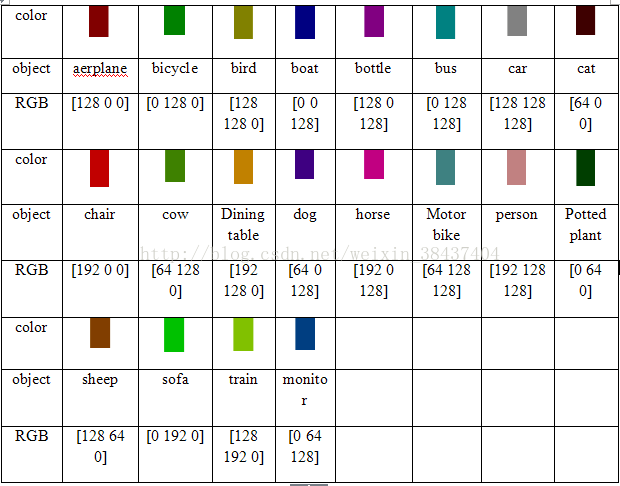reference: https://blog.csdn.net/weixin_38437404/article/details/78788250

## 目标检测中有些代码在训练时会将模型的 BN 层给冻结，这是为什么？

    def freeze_bn(self):
'''Freeze BatchNorm layers.'''
for layer in self.modules():
if isinstance(layer, nn.BatchNorm2d):
layer.eval()


It’s a common practice to freeze BN while training object detection models. This is done because you usually start from some Imagenet pre-trained weights which were trained with large BS (like 256 or larger) while in object detection BS is much smaller. You don’t want to ruin good pretrained statistics so freezing BN is a good idea

https://github.com/yhenon/pytorch-retinanet/issues/151

## 各种卷积

### 空洞卷积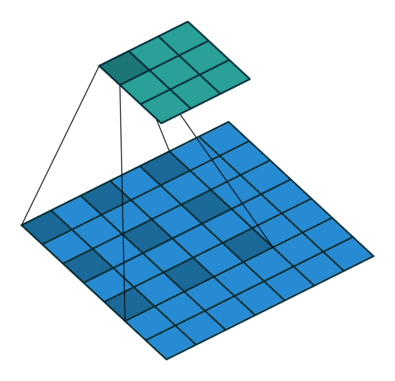reference: https://mp.weixin.qq.com/s/LO1W2saWslf6Ybw_MZAuQQ

### 转置卷积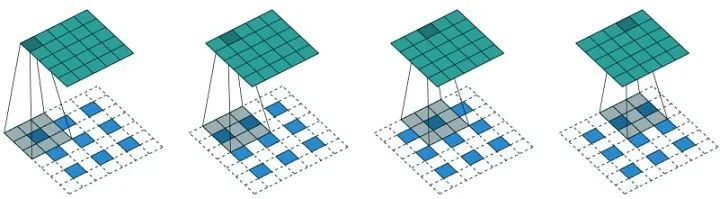## loss 出现 NaN 怎么解决

NaN 可以看成是无穷大或者无穷小，经常是由于在网络计算 loss 的时候除以 0 或者 log(0) 导致的，如果是这种原因的话就得检查代码是否正确，一般在 loss 除以正样本的时候会加个 eps=0.0001 来防止出现 NaN，但如果代码没有问题的话可能就是梯度爆炸了，变得很大，这时候要么就做个梯度裁剪（梯度大于某个值就不要了），或者降低学习率让网络每一步更新的梯度不要太夸张

## matting 跟 segmentation 有什么区别

segmentation 就是对图片中的每一个像素进行分类，判断其所属概率最大的类别。抠图比分割更加严格， 在抠图中有一个公式 I = αF + (1-α)B。我们需要是把 α（不透明度）、F（前景色）和B（背景色）三个变量给解出来。和其它图片进行融合的时候，就会用到 α（不透明度），它可以使得融合效果特别自然，就像 PS 的羽化边缘。

## mAP 计算公式

mmdetection 中，将结果送去计算 mAP 之前，先调用了 bbox_head 的 get_bbox，这个函数默认用了 test_cfg 中的 nms_cfg，也就是会先将得到的 bbox 进行一个类间的 nms 筛除掉一些重复的框再送入 evaluator

## SoftNMS 的优缺点

NMS 直接将和得分最大的 box 的 IOU 大于某个阈值的 box 的得分置零，很粗暴，Soft NMS 就是用稍低一点的分数来代替原有的分数，而不是直接置零

Soft-NMS 具有与传统NMS 相同的算法复杂度，使用高效

Soft-NMS 不需要额外的训练，并易于实现，它可以轻松的被集成到任何物体检测流程中

## Corner Pooling 的作用

##模型压缩的主要方法有哪些

1. 从模型结构上来说分为：模型剪枝，模型蒸馏，NAS 自动学习模型结构等.
2. 模型参数量化上包括数值精度量化到 FP16 等.

## IOU-aware 的思想是什么

IOU-aware 的思想就是在一阶段检测器进行检测时，头部的回归分支和分类分支经常是单独计算的，两者并没有什么关联性，也就是说当一个 anchor 的置信度很高时，网络并不知道他回归的好不好，也就是不知道这个框的质量。因此，就如果我们能将置信度和框的质量相联系起来的话就可以得到更好的结果。这就是 IOU-aware 的由来，具体思想就是在回归分支上再加一个 IOU 分之来预测当前 prediction 和 gt 的 IOU （需要经过一个 sigmoid 归一化到 0-1），IOU 分支的标签就是当前位置 anchor 和相对应的 gt 的 IOU，作者在论文中使用的是 BCE loss。mmdet 中的实现如下：

pred_box = delta2bbox(level_anchor, bbox_pred, self.target_means, self.target_stds)
# the negatives will stores the anchors information(x, y, w, h)
target_box = delta2bbox(level_anchor, bbox_targets, self.target_means, self.target_stds)
iou = bbox_overlaps(target_box, pred_box, is_aligned=True) # (batch*width_i*height_i*A)
iou_pred = iou_pred.permute(0, 2, 3, 1).reshape(-1) # (batch*width_i*height_i*A)
loss_iou = weight_iou*weighted_iou_regression_loss(iou_pred, iou, bbox_weight, avg_factor=num_total_samples)## 各种算法对正负样本的定义是怎样的

TODO，加上 PAA，autoassign，以及最新的一些 cost function 的算法

(1) faster rcnn和retinanet系列采用通用的iou阈值来区分正负样本,不够鲁棒

(2) dynamic rcnn通过在训练中自适应的提高iou阈值来动态改变正负样本定义，算是一个不错的改进

(3) fcos采用的是回归范围限制和中心扩展系数超参来区分正负样本，超参难以设置

(4) atss提出了基于每层候选anchor，然后计算均值和方差来自适应的计算正负样本，虽然超参就一个但是其实有先验即物体越靠近中心越有可能是正样本，没有做到基于预测值进行自适应功能

(5) yolov3和v4系列采用了iou和网格约束先验来区分正负样本，而v5创新性的采用了宽高比例和邻近网格约束进行区分

## 2022.3.10 更新面经题目

 https://leetcode-cn.com/circle/discuss/QmTRU4/

TODO

## 空洞卷积存在的问题？https://blog.csdn.net/chen_Huiling/article/details/112632740

## torch.jit.trace 和 torch.jit.script 的区别

1. trace 只记录走过的 tensor 和对 tensor 的操作，不会记录任何控制流信息，如 if 条件句和循环。因为没有记录控制流的另外的路，也没办法对其进行优化。好处是 trace 深度嵌入 python 语言，复用了所有 python 的语法，在计算流中记录数据流。
2. script 会去理解所有的 code，真正像一个编译器一样去进行 lexer、parser、Semantic analusis 的分析「也就是词法分析语法分析句法分析，形成 AST 树，最后再将 AST 树线性化」。script 相当于一个嵌入在 Python/Pytorch 的 DSL，其语法只是 pytorch 语法的子集，这意味着存在一些 op 和语法 script 不支持，这样在编译的时候就会遇到问题。此外，script 的编译优化方式更像是 CPU 上的传统编译优化，重点对于图进行硬件无关优化，并对 if、loop 这样的 statement 进行优化。

https://zhuanlan.zhihu.com/p/410507557

## Conv BN ReLU 怎样融合### 融合方案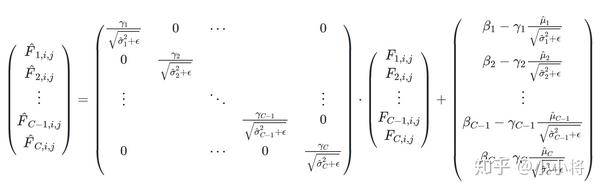•为BN的参数,
•是BN前面Conv层的参数,
•为Conv层的输入，
•为输入层的channel数，
•是Conv层的卷积核大小• filter weights:• bias:def fuse(conv, bn):

fused = torch.nn.Conv2d(
conv.in_channels,
conv.out_channels,
kernel_size=conv.kernel_size,
stride=conv.stride,
bias=True
)

# setting weights
w_conv = conv.weight.clone().view(conv.out_channels, -1)
w_bn = torch.diag(bn.weight.div(torch.sqrt(bn.eps+bn.running_var)))
fused.weight.copy_( torch.mm(w_bn, w_conv).view(fused.weight.size()) )

# setting bias
if conv.bias is not None:
b_conv = conv.bias
else:
b_conv = torch.zeros( conv.weight.size(0) )
b_conv = torch.mm(w_bn, b_conv.view(-1, 1)).view(-1)
b_bn = bn.bias - bn.weight.mul(bn.running_mean).div(
torch.sqrt(bn.running_var + bn.eps)
)
fused.bias.copy_( b_conv + b_bn )

return fused


https://zhuanlan.zhihu.com/p/110552861

## 移动端模型压缩部署有哪些方法

1. 剪枝蒸馏
2. 使用 trt、ncnn 等框架
3. 量化（FP16，INT8）

https://github.com/scutan90/DeepLearning-500-questions/blob/master/ch17%E6%A8%A1%E5%9E%8B%E5%8E%8B%E7%BC%A9%E3%80%81%E5%8A%A0%E9%80%9F%E5%8F%8A%E7%A7%BB%E5%8A%A8%E7%AB%AF%E9%83%A8%E7%BD%B2/%E7%AC%AC%E5%8D%81%E4%B8%83%E7%AB%A0%E6%A8%A1%E5%9E%8B%E5%8E%8B%E7%BC%A9%E3%80%81%E5%8A%A0%E9%80%9F%E5%8F%8A%E7%A7%BB%E5%8A%A8%E7%AB%AF%E9%83%A8%E7%BD%B2.md

## python 中 array 和 list 的区别

list 可以存放不同类型的数据，array 只能存放相同类型的数据

## 10000 类的分类问题应该怎么做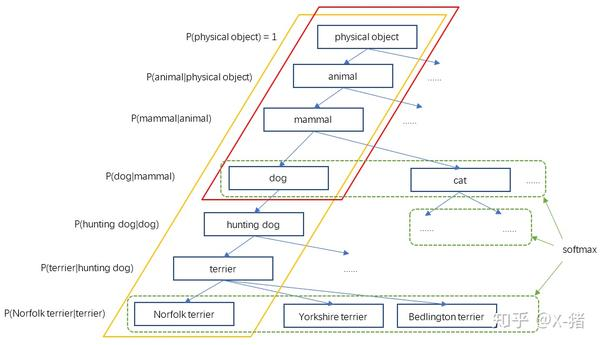## 🌟手撸深度学习算子 / 传统图像处理

### sobel 算子 (jeffin)

# 手动卷积 (Sobel算子)
import cv2
import matplotlib.pyplot as plt
import numpy as np

img = cv2.resize(img, (256,256), cv2.INTER_LINEAR)
cv2.imshow('test', img)

def sobel_x(img):
h, w = img.shape
Newimg_X = np.zeros_like(img)
sobel_X = np.array([[-1, 0, 1], [-2, 0, 2], [-1, 0, 1]])
for j in range(w-2):
for i in range(h-2):
Newimg_X[i+1, j+1] = abs(np.sum(img[i:i+3, j:j+3] * sobel_X))
return np.uint8(Newimg_X)

def sobel_y(img):
h, w = img.shape
Newimg_Y = np.zeros_like(img)
sobel_Y = np.array([[-1, -2, -1], [0, 0, 0], [1, 2, 1]])
for j in range(w-2):
for i in range(h-2):
Newimg_Y[i+1, j+1] = abs(np.sum(img[i:i+3, j:j+3] * sobel_Y))
return np.uint8(Newimg_Y)


### 直方图均衡化

img = cv2.imread('imgs/wild.jpeg', 0)  # 1. 读取灰度图

prob = np.array([np.sum(img == i) for i in range(256)])
r, c = img.shape
# 2. 得到每一个像素出现的概率
prob = prob / (r * c)

plot(prob, "原图直方图")

# 3. 直方图均衡化
prob = np.cumsum(prob)  # 3.1 累计概率

img_map = [int(i * prob[i]) for i in range(256)]  # 3.2 像素值映射(要记住！)
# 3.3 像素值替换
for ri in range(r):
for ci in range(c):
img[ri, ci] = img_map[img[ri, ci]]

prob = np.array([np.sum(img == i) for i in range(256)])
r, c = img.shape
prob = prob / (r * c)
plot(prob, "直方图均衡化结果")
plt.figure()
plt.imshow(cv2.cvtColor(img, cv2.COLOR_BGR2RGB))
plt.show()### 求 Tensor 每个像素与其他像素之间的自相似度

# 计算 2D 或者 4D tensor 相似度
import torch

def sim(query, key, eps = 1e-8):
input_dim = query.dim()
assert query.dim() == key.dim()
if (input_dim == 4):
B, C, H, W = query.shape
query = query.reshape(B, C, -1) # B,C, H*W
# 用 permute 会出错，因为只是转置一部分
query = F.normalize(query, p=2, dim=1, eps=eps).transpose(1,2) # B, H*W, C
key = key.reshape(B, C, -1) # B, C, H*W
key = F.normalize(key, p=2, dim=1, eps=eps)
similarity = torch.matmul(query, key) # B, H*W, H*W
assert similarity.max() < 1.001 and similarity.min() > -1.001, 'cosine similarity ranges [-1, 1]'
return similarity
elif input_dim == 2:
B, C = query.shape
# 用 permute 会出错，因为只是转置一部分
query = F.normalize(query, p=2, dim=1, eps=eps) # B, C
key = key.permute(1, 0) # C, B
key = F.normalize(key, p=2, dim=0, eps=eps)
similarity = torch.matmul(query, key) # B, B
assert similarity.max() < 1.001 and similarity.min() > -1.001, 'cosine similarity ranges [-1, 1]'
return similarity

input = torch.randn(16, 256, 8, 8)
print(sim(input, input).shape)
input = torch.randn(16, 256)
print(sim(input, input).shape)


### 用 SGD 或者 Adam 求解方程

SGD 解方程

def cal_dx(func,x,eps=1e-8):
d_func = func(x+eps) - func(x)
dx = d_func / eps
return dx

def solve(func,x,loss_func=lambda x:x**2, lr=0.001,num_iters=60000):
print('init x:', x)
for i in range(num_iters):
y = func(x)
d_loss_d_y = cal_dx(loss_func, y)
dy_dx = cal_dx(func, x)
return x

def check(func,x,eps=1e-8):
if abs(func(x)) < eps:
print('done')
else:
print('failed')

if __name__ == '__main__':
init = 7
func = lambda x: x**2+2*x-24
x = solve(func,init)
print(x)
check(func,x)


SGDm (把 solve 函数换一下)

def solve(func,x,loss_func=lambda x:x**2, lr=0.001,num_iters=60000, momentum=0.99):
print('init x:', x)
momentum_buf = None
for i in range(num_iters):
y = func(x)
d_loss_d_y = cal_dx(loss_func, y)
dy_dx = cal_dx(func, x)
if momentum_buf:
else:
x = x -


### BN (BatchNorm 在 train 和 test 的时候有什么不同)


import torch
from torch import nn

class BatchNorm(nn.Module):
# num_features：完全连接层的输出数量或卷积层的输出通道数。
# num_dims：2表示完全连接层，4表示卷积层
def __init__(self, num_features, num_dims):
super().__init__()
if num_dims == 2:
shape = (1, num_features)
else:
shape = (1, num_features, 1, 1)
# 参与求梯度和迭代的拉伸和偏移参数，分别初始化成1和0
self.gamma = nn.Parameter(torch.ones(shape))
self.beta = nn.Parameter(torch.zeros(shape))
# 非模型参数的变量初始化为0和1
self.moving_mean = torch.zeros(shape)
self.moving_var = torch.ones(shape)

def forward(self, X):
# 如果X不在内存上，将moving_mean和moving_var
# 复制到X所在显存上
if self.moving_mean.device != X.device:
self.moving_mean = self.moving_mean.to(X.device)
self.moving_var = self.moving_var.to(X.device)
# 保存更新过的moving_mean和moving_var
Y, self.moving_mean, self.moving_var = batch_norm(
X, self.gamma, self.beta, self.moving_mean,
self.moving_var, eps=1e-5, momentum=0.9)
return Y

def batch_norm(X, gamma, beta, moving_mean, moving_var, eps, momentum):
# 如果是在预测模式下，直接使用传入的移动平均所得的均值和方差
X_hat = (X - moving_mean) / torch.sqrt(moving_var + eps)
else:
assert len(X.shape) in (2, 4)
if len(X.shape) == 2:
# 使用全连接层的情况，计算特征维上的均值和方差
mean = X.mean(dim=0)
var = ((X - mean) ** 2).mean(dim=0)
else:
# 使用二维卷积层的情况，计算通道维上（axis=1）的均值和方差。
# 这里我们需要保持X的形状以便后面可以做广播运算
mean = X.mean(dim=(0, 2, 3), keepdim=True)
var = ((X - mean) ** 2).mean(dim=(0, 2, 3), keepdim=True)
# 训练模式下，用当前的均值和方差做标准化
X_hat = (X - mean) / torch.sqrt(var + eps)
# 更新移动平均的均值和方差
moving_mean = momentum * moving_mean + (1.0 - momentum) * mean
moving_var = momentum * moving_var + (1.0 - momentum) * var
Y = gamma * X_hat + beta  # 缩放和移位
return Y, moving_mean.data, moving_var.data


### 手写 MaxPooling / AvgPooling

import numpy as np
import torch
class MaxPooling2D:
def __init__(self, kernel_size=(2, 2), stride=2):
self.kernel_size = kernel_size
self.w_height = kernel_size
self.w_width = kernel_size

self.stride = stride

self.x = None
self.in_height = None
self.in_width = None

self.out_height = None
self.out_width = None
# 要记录下在当前的滑动窗中最大值的索引，反向求导要用到
self.arg_max = None

def __call__(self, x):
self.x = x
self.in_height = np.shape(x)
self.in_width = np.shape(x)

self.out_height = int((self.in_height - self.w_height) / self.stride) + 1
self.out_width = int((self.in_width - self.w_width) / self.stride) + 1

out = np.zeros((self.out_height, self.out_width))
self.arg_max = np.zeros_like(out, dtype=np.int32)

for i in range(self.out_height):
for j in range(self.out_width):
start_i = i * self.stride
start_j = j * self.stride
end_i = start_i + self.w_height
end_j = start_j + self.w_width
out[i, j] = np.max(x[start_i: end_i, start_j: end_j])
self.arg_max[i, j] = np.argmax(x[start_i: end_i, start_j: end_j])

self.arg_max = self.arg_max
return out

def backward(self, d_loss):
dx = np.zeros_like(self.x)
for i in range(self.out_height):
for j in range(self.out_width):
start_i = i * self.stride
start_j = j * self.stride
end_i = start_i + self.w_height
end_j = start_j + self.w_width
# 将索引展开成二维的
index = np.unravel_index(self.arg_max[i, j], self.kernel_size)
dx[start_i:end_i, start_j:end_j][index] = d_loss[i, j] #
return dx

class AvgPooling2D:
def __init__(self, kernel_size=(2, 2), stride=2):
self.stride = stride
self.kernel_size = kernel_size
self.w_height = kernel_size
self.w_width = kernel_size

def __call__(self, x):
self.x = x
self.in_height = x.shape
self.in_width = x.shape

self.out_height = int((self.in_height - self.w_height) / self.stride) + 1
self.out_width = int((self.in_width - self.w_width) / self.stride) + 1
out = np.zeros((self.out_height, self.out_width))

for i in range(self.out_height):
for j in range(self.out_width):
start_i = i * self.stride
start_j = j * self.stride
end_i = start_i + self.w_height
end_j = start_j + self.w_width
out[i, j] = np.mean(x[start_i: end_i, start_j: end_j])
return out

def backward(self, d_loss):
dx = np.zeros_like(self.x)

for i in range(self.out_height):
for j in range(self.out_width):
start_i = i * self.stride
start_j = j * self.stride
end_i = start_i + self.w_height
end_j = start_j + self.w_width
# 直接将 loss 均分给每一个像素点
dx[start_i: end_i, start_j: end_j] = d_loss[i, j] / (self.w_width * self.w_height)
return dx

np.set_printoptions(precision=4, suppress=True, linewidth=120)
x_numpy = np.random.random((1, 1, 6, 9))

max_pool_tensor = torch.nn.AvgPool2d((2, 2), 2)
max_pool_numpy = AvgPooling2D((2, 2), stride=2)

out_numpy = max_pool_numpy(x_numpy[0, 0])
out_tensor = max_pool_tensor(x_tensor)

d_loss_numpy = np.random.random(out_tensor.shape)
out_tensor.backward(d_loss_tensor)

dx_numpy = max_pool_numpy.backward(d_loss_numpy[0, 0])
# print('input \n', x_numpy)
print("out_numpy \n", out_numpy)
print("out_tensor \n", out_tensor.data.numpy())

print("dx_numpy \n", dx_numpy)
print("dx_tensor \n", dx_tensor.data.numpy())


### 手撸IOU计算公式

def bb_intersection_over_union(boxA, boxB):
boxA = [int(x) for x in boxA]
boxB = [int(x) for x in boxB]

xA = max(boxA, boxB)
yA = max(boxA, boxB)
xB = min(boxA, boxB)
yB = min(boxA, boxB)

# 要加上 1 ，因为像素是一个点，并不是一个矩形
interArea = max(0, xB - xA + 1) * max(0, yB - yA + 1)

boxAArea = (boxA - boxA + 1) * (boxA - boxA + 1)
boxBArea = (boxB - boxB + 1) * (boxB - boxB + 1)

iou = interArea / float(boxAArea + boxBArea - interArea)

return iou


def bbox_overlaps(bboxes1, bboxes2, mode='iou', is_aligned=False, eps=1e-6):

assert mode in ['iou', 'iof', 'giou'], f'Unsupported mode {mode}'
# Either the boxes are empty or the length of boxes' last dimension is 4
assert (bboxes1.size(-1) == 4 or bboxes1.size(0) == 0)
assert (bboxes2.size(-1) == 4 or bboxes2.size(0) == 0)

# Batch dim must be the same
# Batch dim: (B1, B2, ... Bn)
assert bboxes1.shape[:-2] == bboxes2.shape[:-2]
batch_shape = bboxes1.shape[:-2]

rows = bboxes1.size(-2)
cols = bboxes2.size(-2)
if is_aligned:
assert rows == cols

area1 = (bboxes1[..., 2] - bboxes1[..., 0]) * (
bboxes1[..., 3] - bboxes1[..., 1])
area2 = (bboxes2[..., 2] - bboxes2[..., 0]) * (
bboxes2[..., 3] - bboxes2[..., 1])

if is_aligned:
lt = torch.max(bboxes1[..., :2], bboxes2[..., :2])  # [B, rows, 2]
rb = torch.min(bboxes1[..., 2:], bboxes2[..., 2:])  # [B, rows, 2]

wh = fp16_clamp(rb - lt, min=0)
overlap = wh[..., 0] * wh[..., 1]

union = area1 + area2 - overlap
else:
lt = torch.max(bboxes1[..., :, None, :2],
bboxes2[..., None, :, :2])  # [B, rows, cols, 2]
rb = torch.min(bboxes1[..., :, None, 2:],
bboxes2[..., None, :, 2:])  # [B, rows, cols, 2]

wh = fp16_clamp(rb - lt, min=0)
overlap = wh[..., 0] * wh[..., 1]

union = area1[..., None] + area2[..., None, :] - overlap

eps = union.new_tensor([eps])
union = torch.max(union, eps)
ious = overlap / union
return ious


### 手撸 NMS (NMS 怎样实现的)

NMS 算法的主要流程如下所示：

1. 先标记最大概率矩形框 F 是我们要保留下来的；
2. 从最大概率矩形框 F 开始，分别判断 A~E 与 F 的重叠度 IOU（两框的交并比）是否大于某个设定的阈值，假设 B、D 与 F 的重叠度超过阈值，那么就扔掉 B、D；
3. 从剩下的矩形框 A、C、E 中，选择概率最大的 E，标记为要保留下来的，然后判读 E 与 A、C 的重叠度，扔掉重叠度超过设定阈值的矩形框

import numpy as np

def py_nms(dets, thresh):
"""Pure Python NMS baseline."""
#x1、y1、x2、y2、以及score赋值
x1 = dets[:, 0]
y1 = dets[:, 1]
x2 = dets[:, 2]
y2 = dets[:, 3]
scores = dets[:, 4]

#每一个候选框的面积
areas = (x2 - x1 + 1) * (y2 - y1 + 1)
#order是按照score降序排序的
order = scores.argsort()[::-1]

keep = []
while order.size > 0:
i = order
keep.append(i)
xx1 = np.maximum(x1[i], x1[order[1:]])
yy1 = np.maximum(y1[i], y1[order[1:]])
xx2 = np.minimum(x2[i], x2[order[1:]])
yy2 = np.minimum(y2[i], y2[order[1:]])

#计算相交框的面积,注意矩形框不相交时w或h算出来会是负数，用0代替
w = np.maximum(0.0, xx2 - xx1 + 1)
h = np.maximum(0.0, yy2 - yy1 + 1)
inter = w * h
#计算重叠度IOU：重叠面积/（面积1+面积2-重叠面积）
ovr = inter / (areas[i] + areas[order[1:]] - inter)

#找到重叠度不高于阈值的矩形框索引
inds = np.where(ovr <= thresh)
#将order序列更新，由于前面得到的矩形框索引要比矩形框在原order序列中的索引小1，所以要把这个1加回来
order = order[inds + 1]
return keep

# test
if __name__ == "__main__":
dets = np.array([[30, 20, 230, 200, 1],
[50, 50, 260, 220, 0.9],
[210, 30, 420, 5, 0.8],
[430, 280, 460, 360, 0.7]])
thresh = 0.35
keep_dets = py_nms(dets, thresh)
print(keep_dets)
print(dets[keep_dets])


reference:

https://github.com/kuaikuaikim/DFace/

https://blog.csdn.net/Blateyang/article/details/79113030

## 🌟Leetcode 题目框架

### 通用排序算法

class Solution {
int sort(vector<int>& nums,int left,int right)
{
int pivot=rand()%(right-left+1)+left;
int temp=nums[left];
nums[left]=nums[pivot];
nums[pivot]=temp;
pivot=nums[left];
while(left<right)
{
while(left<right&&nums[right]>=pivot)
right--;
nums[left]=nums[right];
while(left<right&&nums[left]<=pivot)
left++;
nums[right]=nums[left];
}
nums[left]=pivot;
return left;
}
void quick_sort(vector<int>& nums,int left,int right)
{
if(left>=right)
return;
int mid=sort(nums,left,right);
quick_sort(nums,left,mid-1);
quick_sort(nums,mid+1,right);
}
public:
vector<int> sortArray(vector<int>& nums) {
quick_sort(nums,0,nums.size()-1);
return nums;
}
};


class Solution {
void merge(vector<int>& nums,int left,int right,vector<int>& ans)
{
int mid=(right-left)/2+left;
int i=left,j=mid+1,k=0;
while(i<=mid&&j<=right)
{
if(nums[i]<=nums[j])
ans[k++]=nums[i++];
else ans[k++]=nums[j++];
}
while(i<=mid)
{
ans[k++]=nums[i++];
}
while(j<=right)
ans[k++]=nums[j++];
for(int i=left;i<=right;i++)
nums[i]=ans[i-left];
}
void mergeSort(vector<int>& nums,int left,int right,vector<int>& ans)
{
if(left>=right)
return ;
int mid=(right-left)/2+left;
mergeSort(nums,left,mid,ans);
mergeSort(nums,mid+1,right,ans);
merge(nums,left,right,ans);
}
public:
vector<int> sortArray(vector<int>& nums) {
vector <int> ans(nums.size());
mergeSort(nums,0,nums.size()-1,ans);
return nums;
}
};


class Solution {
{
while(i<size)
{
int left=2*i+1,right=2*i+2;
int max=i;
if(left<size&&nums[left]>nums[max])
max=left;
if(right<size&&nums[right]>nums[max])
max=right;
if(max==i)
break;
swap(nums[i],nums[max]);
i=max;
}
}
void maxHeapity(vector<int>& nums,int size)
{
for(int i=size/2-1;i>=0;i--)
}
public:
vector<int> sortArray(vector<int>& nums) {
int n=nums.size();
maxHeapity(nums,n);
swap(nums,nums[n-1]);
for(int i=n-2;i>0;i--)
{
n--;
swap(nums,nums[i]);
}
return nums;
}
};


### 二分框架（有序数组，找值或者左右边界）

class Solution {
public:
// 二分正确版本
vector<int> searchRange(vector<int>& nums, int target) {
vector<int> res(2, -1);
if (!nums.size()) {
return res;
}
int left = begin(nums, target);
int right = end(nums, target);
res = {left, right};
return res;
}
int begin(vector<int> & nums, int target) {
int left = 0, right = nums.size() - 1;
while (left <= right) {
// 防止溢出
int mid = left + (right - left) / 2;
if (nums[mid] < target) {
left = mid + 1;
} else if (nums[mid] > target) {
right = mid - 1;
} else if (nums[mid] == target) {
// 别返回，锁定左侧边界
right = mid - 1;
}
}
if (left >= nums.size() || nums[left] != target) {
return -1;
}
return left;
}

int end(vector<int> & nums, int target) {
int left = 0, right = nums.size() - 1;
while (left <= right) {
int mid = left + (right - left) / 2;
if (nums[mid] < target) {
left = mid + 1;
} else if (nums[mid] > target) {
right = mid - 1;
} else if (nums[mid] == target) {
// 别返回，锁定右侧边界
left = mid + 1;
}
}
if (right < 0 || nums[right] != target) {
return -1;
}
return right;
}
};


### 链表相交 (双指针)

class Solution {
public:
return NULL;
}
// 不相交的话最后都是 null， 也能跳出来
while (p1 != p2) {
p1 = p1 ? p1->next : headB;
p2 = p2 ? p2->next : headA;
}
return p1;
}
};


### 合并 k 个有序链表 (优先队列，堆)

// 自定义一个比较函数传入优先队列的构造函数
class cmp1
{
public:
bool operator() (ListNode * a, ListNode * b)
{
// 小顶堆保证堆顶元素与其他元素比较时， < 一定是 false，所以这里是 >
return a->val > b->val;
}
};

class Solution
{
public:

ListNode* mergeKLists(vector<ListNode*>& lists)
{
if (lists.size() == 0)
return NULL;
// 优先队列的构造函数
priority_queue<ListNode *, vector<ListNode*>, cmp1> minHeap;
for (ListNode * x : lists)
{
if (x)
// 将存在值的链表 push 进小顶堆
minHeap.push(x);
}

ListNode * dummy = new ListNode(0);
ListNode * x = dummy;
while (!minHeap.empty())
{
// 找到堆中最小的值
ListNode * y = minHeap.top();
minHeap.pop();
// 更新结果链表
x->next = y;
x = x->next;
// 将这个链表下一个值再 push 进去进行排序
if (y->next)
{
minHeap.push(y->next);
}
}
return dummy->next;

}
};


### 环形链表2 (双指针判断交点)

class Solution {
public:
return NULL;
}
while (fast && fast->next) {
slow = slow->next;
fast = fast->next->next;
if (slow == fast) {
break;
}
}
if (!fast || !fast->next) {
// 无环
return NULL;
}
while (fast != slow) {
fast = fast->next;
slow = slow->next;
}
return slow;
}
};


### 分割等和子集

// 转化成背包问题来做，数字能否填满 sum/2 容量的背包

class Solution {
public:
bool canPartition(vector<int>& nums) {
int sum = 0;
for (int i : nums) sum += i;
// 为奇数的话不能分割
if (sum % 2) return false;
int target = sum / 2;
// 定义 DP table
int size = nums.size();
// DP table 的定义：dp[i][j]->对于前i个物体，当背包的容量为j时，能不能装满
vector<vector<int>> dp(size+1, vector<int>(target+1, false));
for (int i = 0; i <= size; i++) {
dp[i] = true;
}
for (int j = 0; j <= target; j++) {
dp[j] = false;
}
for (int i = 1; i <= size; i++) {
for (int j = 1; j <= target; j++) {
// 背包容量不足，不足以放进第 i 个物体
if (j-nums[i-1] < 0) {
dp[i][j] = dp[i-1][j];
} else {
// 将低第 i 个数放不放进背包
dp[i][j] = dp[i-1][j] || dp[i-1][j-nums[i-1]];
}
}
}
return dp[size][target];
}
};


### 股票问题

int maxProfit(vector<int>& prices) {
// 股票问题，三层 dp
// dp[i][k][0/1] 表示第i天，最多进行k次交易时，持有或者不持有股票的最大利润
// 状态转移方程：
// dp[i][k] = max(dp[i-1][k], dp[i-1][k] + prices[i])
// dp[i][k] = max(dp[i-1][k], dp[i-1][k-1] - prices[i])

int k_max = 2;
vector<vector<vector<int>>> dp(prices.size(), vector<vector<int>>(k_max+1, vector<int>(2)));
for (int i = 0; i < prices.size(); i++) {
// 穷举所有 k
for (int k = 1; k <= k_max; k++) {
if (i == 0) {
// base case
// dp[-1] = 0, dp[-1] = -inf
dp[i][k] = 0;
dp[i][k] = -prices[i];
continue;   // continue 不要忘记加
}
// 今天不持有股票，利润=max(休息，卖掉)
dp[i][k] = max(dp[i-1][k], dp[i-1][k]+prices[i]);
// 今天持有股票，利润=max(休息，买入)
dp[i][k] = max(dp[i-1][k], dp[i-1][k-1]-prices[i]);
}
}
// 最后一天肯定是把股票卖出去的利润大于不买的利润
return dp[prices.size()-1][k_max];
}


### 打家劫舍问题

// 首尾不能同时偷，所以有三种情况，只偷首，只偷尾，首位都不偷
// 第三种情况的收益没有前两种大，所以只判断两种就行了
int rob(vector<int>& nums) {
if (!nums.size()) {
return 0;
}
if (nums.size() == 1) {
return nums;
}
// 两种情况取最大值
return max(rob_between(nums, 0, nums.size()-1), rob_between(nums, 1, nums.size()));
}
// 打家劫舍第一题的做法
int rob_between(vector<int>& nums, int begin, int end) {
vector<vector<int>> dp(nums.size(), vector<int>(2));
for (int i = begin; i < end; i++) {
if (i == begin) {
dp[i] = 0;
dp[i] = nums[i];
continue;
}
dp[i] = max(dp[i-1], dp[i-1]);
dp[i] = max(dp[i-1]+nums[i], dp[i-1]);
}
cout << dp[end-1];
return max(dp[end-1], dp[end-1]);
}

unordered_map<TreeNode*, int> memo;
int rob(TreeNode* root) {
if (!root) {
return 0;
}
// 备忘录消除重叠子问题
if (memo.find(root) != memo.end()) {
return memo[root];
}
// 抢
int do_it = root->val
+ (root->left ? rob(root->left->left)+rob(root->left->right): 0)
+ (root->right ? rob(root->right->left)+rob(root->right->right): 0);
// 不抢
int not_do = rob(root->left)+rob(root->right);
int res = max(do_it, not_do);
memo[root] = res;
return res;
}


### 接雨水问题

class Solution {
public:
// 双指针
int trap(vector<int>& height) {
int res = 0;
int left = 0, right = height.size()-1;
// 初始化当前左边区域最大的高，右边区域最大的高
int l_max = 0, r_max = 0;
while (left < right) {
l_max = max(l_max, height[left]);
r_max = max(r_max, height[right]);
if (l_max < r_max) {
res += l_max - height[left];
left++;
} else {
res += r_max - height[right];
right--;
}
}
return res;
}
};


### 约瑟夫环

0,1,···,n-1这n个数字排成一个圆圈，从数字0开始，每次从这个圆圈里删除第m个数字（删除后从下一个数字开始计数）。求出这个圆圈里剩下的最后一个数字。

class Solution {
public:
int lastRemaining(int n, int m) {
int res = 0;
// 只有一个数字的话，也即是 i==1 情况，留下的一定是 0
for (int i = 2; i <= n; i++) {
res = (res + m) % i;
}
return res;
}
};


### 封闭岛屿的数量

class Solution {
public:
int closedIsland(vector<vector<int>>& grid) {
int res = 0;
// 先将边缘的岛屿给淹了
for (int i = 0; i < grid.size(); i++) {
dfs(grid, i, 0);
dfs(grid, i, grid.size() - 1);
}
for (int j = 0; j < grid.size(); j++) {
dfs(grid, 0, j);
dfs(grid, grid.size() - 1, j);
}
// 再判断岛屿个数
for (int i = 0; i < grid.size(); i++) {
for (int j = 0; j < grid.size(); j++) {
if (grid[i][j] == 0) {
res++;
dfs(grid, i, j);
}
}
}
return res;
}

void dfs(vector<vector<int>> &grid, int i, int j) {
if ((i >= grid.size() || j >= grid.size() || i < 0 || j < 0)) {
return;
}
if (grid[i][j] == 1) {
return;
}
// 不断把四周淹了
grid[i][j] = 1;
dfs(grid, i-1, j);
dfs(grid, i+1, j);
dfs(grid, i, j-1);
dfs(grid, i, j+1);
}
};


### 最小覆盖子串 (经典滑动窗口)

class Solution {
public:
string minWindow(string s, string t) {
// 滑动窗口框架，左闭右开
int left = 0, right = 0;
unordered_map<char, int> need, window;
int valid_num = 0; // 滑动窗中满足答案的字符串个数
int start = 0; int len = INT_MAX;   // 最终答案在字符串中的起始索引和长度
for (auto & i: t) need[i]++;
while (right < s.size()) {
char cur = s[right];
right++;
// 当前字符是我们需要的
if (need.count(cur)) {
window[cur]++;
// 有一个字符达到个数之后就使 valid_num++
if (window[cur] == need[cur]) {
valid_num++;
}
}
// 所有的字符都达到个数之后就开始收缩窗口
while (valid_num == need.size()) {
if (right - left < len) {
start = left;
len = right - left;
}
char left_cur = s[left];
left++;
if (need.count(left_cur)) {
if (window[left_cur] == need[left_cur]) {
valid_num--;
}
// window 缩减要放在后面，不然会对 valid 的结果产生影响
// 其实 left 和 right 做的事情是对称的
window[left_cur]--;
}
}
}
return len == INT_MAX ? string() : s.substr(start, len);
}
};


### 最长公共子序列 (动规)

// string 类题目就用两个指针指向两个 string，一边移动，动态规划题目
class Solution {
public:
int longestCommonSubsequence(string text1, string text2) {
int l1 = text1.size();
int l2 = text2.size();
// 定义：s1[0..i-1] 和 s2[0..j-1] 的 lcs 长度为 dp[i][j]
vector<vector<int>> dp(l1+1, vector<int>(l2+1, 0));

for (int i = 1; i <= l1; i++) {
for (int j = 1; j <= l2; j++) {
if (text1[i-1] == text2[j-1]) {
dp[i][j] = 1 + dp[i-1][j-1];
} else {
dp[i][j] = max(dp[i][j-1], dp[i-1][j]);
}
}
}
return dp[l1][l2];
}
};


### 最长回文子序列 (动规)

// 更绝的做法是将 s 反转，求他们的最长公共子序列就是答案

class Solution {
public:
int longestPalindromeSubseq(string s) {
int n = s.size();
// dp 数组全部初始化为 0
// dp[i][j] 代表了 s[i,j] 的最长回文子串
vector<vector<int>> dp(n, vector<int>(n, 0));
// base case，i 和 j 相等的话回文串长度就是 1，也就是自己
// 并且 i > j 的地方，长度为 0，已经被初始化了
for (int i = 0; i < n; i++)
dp[i][i] = 1;
// 反着遍历保证正确的状态转移
for (int i = n - 1; i >= 0; i--) {
for (int j = i + 1; j < n; j++) {
// 状态转移方程
if (s[i] == s[j])
dp[i][j] = dp[i + 1][j - 1] + 2;
else
dp[i][j] = max(dp[i + 1][j], dp[i][j - 1]);
}
}
// 整个 s 的最长回文子串长度
return dp[n - 1];

}
};


### 最长递增子序列 (动规)

class Solution {
public:
int lengthOfLIS(vector<int>& nums) {
vector<int> dp(nums.size(), 1);
// 对每一个数字来说
// dp[i] 代表了以它结尾的最长序列长度
// 计算的逻辑就是前面以比他的值小的数为结尾的最长序列长度 + 1，由于可能有很多个，所以取最大值
for (int i = 0; i < nums.size(); i++) {
for (int j = 0; j < i; j++) {
if (nums[i] > nums[j]) {
dp[i] = max(dp[i], dp[j] + 1);
}
}
}

// 找到最大的序列
int res = 0;
for (int i = 0; i < dp.size(); i++) {
if (dp[i] > res) {
res = dp[i];
}
}
return res;
}
};


### 爬楼梯 /斐波那契 (动规)

//假设你正在爬楼梯。需要 n 阶你才能到达楼顶。
//每次你可以爬 1 或 2 个台阶。你有多少种不同的方法可以爬到楼顶呢？
class Solution {
public:
int climbStairs(int n) {
vector<int> dp(n+1);
dp = 1;
dp = 1;
for (int i = 2; i <= n; i++) {
// 爬到第n阶的种数=爬到n-1阶的种数+爬到n-2阶的种数
// 因为两种情况下都只用再爬1次或者2次
dp[i] = dp[i-1] + dp[i-2];
}
return dp[n];
}
};


### 零钱兑换 (动规)

// 动态规划，凑 amount 需要的硬币数等于凑 amount -coin 需要的硬币数 + 1
class Solution {
public:
int coinChange(vector<int>& coins, int amount) {
// 不能初始化为 INT_MAX，否则会越界
vector<int> dp(amount + 1, amount + 1);
// base case
dp = 0;
for (int i = 1; i <= amount; i++) {
for (int c: coins) {
// < 0 的话这种面值就不行
if (i - c < 0) {
continue;
} else {
dp[i] = min(dp[i], dp[i - c] + 1);
}
}
}
return dp[amount] == amount + 1 ? -1 : dp[amount];
}
};


### 零钱兑换 II (背包动规)

class Solution {
public:
int change(int amount, vector<int>& coins) {

// 定义 DP table
int size = coins.size();
// DP table 的定义：若只使用前 i 个物品（可以重复使用），当背包容量为 j 时，有 dp[i][j] 种方法可以装满背包
vector<vector<int>> dp(size+1, vector<int>(amount+1, 0));
// 容量为 0，只有一种（什么都不装）
for (int i = 0; i <= size; i++) {
dp[i] = 1;
}

for (int i = 1; i <= size; i++) {
for (int j = 1; j <= amount; j++) {
// 背包容量不足，不足以使用第 i 个硬币
if (j-coins[i-1] < 0) {
dp[i][j] = dp[i-1][j];
} else {
// 将低第 i 个数放不放进背包
// dp[i][j-coins[i-1]] 也就代表放进背包，意思就是已知第 i 个硬币的面值
// 使用前 i -1 种硬币能凑出总额减去自己面额的组合数，但是这里硬币无限用
// 所以反映到 dp 方程上就是 i 而不是 i-1
dp[i][j] = dp[i-1][j] + dp[i][j-coins[i-1]];
}
}
}
return dp[size][amount];
}
};


### 正则表达式 (TODO)

class Solution {
public:
bool isMatch(string s, string p) {
int m = s.size() + 1, n = p.size() + 1;
vector<vector<bool>> dp(m, vector<bool>(n, false));
dp = true;
// 初始化首行
for(int j = 2; j < n; j += 2)
dp[j] = dp[j - 2] && p[j - 1] == '*';
// 状态转移
for(int i = 1; i < m; i++) {
for(int j = 1; j < n; j++) {
if(p[j - 1] == '*') {
if(dp[i][j - 2]) dp[i][j] = true;                              // 1.
else if(dp[i - 1][j] && s[i - 1] == p[j - 2]) dp[i][j] = true; // 2.
else if(dp[i - 1][j] && p[j - 2] == '.') dp[i][j] = true;      // 3.
} else {
if(dp[i - 1][j - 1] && s[i - 1] == p[j - 1]) dp[i][j] = true;  // 1.
else if(dp[i - 1][j - 1] && p[j - 1] == '.') dp[i][j] = true;  // 2.
}
}
}
return dp[m - 1][n - 1];
}
};



### 最长回文子串 (双指针中心开花)

class Solution {
public:
string longestPalindrome(string s) {
// 回文串可能是单数可能是双数
string res;
for (int i = 0; i < s.size(); i++) {
string s_single = hwstring(s, i, i);
string s_double = hwstring(s, i, i+1);
res = res.size() > s_single.size() ? res : s_single;
res = res.size() > s_double.size() ? res : s_double;
}
return res;
}

string hwstring(string s, int l, int r) {
while (l >= 0 && r < s.size()) {
if (s[l] == s[r]) {
l--;
r++;
} else {
break;
}
}
// start, size
return s.substr(l+1, r-l-1);
}
};


### 下一个更大的元素/每日温度 (单调栈)

class Solution {
public:
vector<int> dailyTemperatures(vector<int>& temperatures) {
stack<int> stk;
vector<int> res(temperatures.size());
// 倒着遍历，这样的话就是 pop 就是正序
for (int i = temperatures.size()-1; i >= 0; i--) {
// 把后面的矮过当前元素的值都给 pop 出来
while (!stk.empty() && temperatures[stk.top()] <= temperatures[i]) {
stk.pop();
}
// 空的时候说明是最后一个，或者后面的元素没有比他大的（之前已经把矮的给 pop 出来了）
res[i] = stk.empty() ? 0 : stk.top()-i;
stk.push(i);
}
return res;
}
};


### rand7() 实现 rand10()

class Solution:
def rand10(self):
while True:
res = (rand7()-1)*7 + rand7()#构造1~49的均匀分布
if res <= 40:#剔除大于40的值，1-40等概率出现。
break
return res%10+1#构造1-10的均匀分布


### 乘积最大的子数组

class Solution {
public:
int maxProduct(vector<int>& nums) {
int res = nums;
int min_val = res, max_val = res;
for (int i = 1; i < nums.size(); i++) {
// 如果数组的数是负数，那么会导致最大的变最小的，最小的变最大的。因此交换两个的值。
if (nums[i] < 0) {
swap(min_val, max_val);
}
max_val = max(max_val * nums[i], nums[i]);
min_val = min(min_val * nums[i], nums[i]);
res = max(max_val, res);
}
return res;
}
};


### K 个一组翻转链表

class Solution {
public:
ListNode* reverseKGroup(ListNode* head, int k) {
return nullptr;
}
for (int i = 0; i < k; i++) {
end = end->next;
}
ListNode *reversed = reverse(begin, end);
// begin 现在成了翻转后链表的最后一个结点
begin->next = reverseKGroup(end, k);
return reversed;
}

// 反转 [A, B) 区间内的结点，注意是左闭右开，相当于把正常的函数签名由 null 改成了 B
ListNode *reverse(ListNode *A, ListNode *B) {
ListNode *pre = nullptr;
ListNode *cur = A;
ListNode *tmp = nullptr;
while (cur != B) { // 相当于把 A != nullptr 改成了 A != B
tmp = cur->next;
cur->next = pre;
pre = cur;
cur = tmp;
}
return pre;
}
};


### 二叉树层序遍历

class Solution {
private:
vector<vector<int>> res;
public:
vector<vector<int>> levelOrder(TreeNode* root) {
if (!root) {
return res;
}
queue<TreeNode*> q;
q.push(root);
while (!q.empty()) {
int size = q.size();
vector<int> vec;
for (int i = 0; i < size; i++) {
TreeNode* cur = q.front();
q.pop();
vec.push_back(cur->val);
if (cur->left) q.push(cur->left);
if (cur->right) q.push(cur->right);
}
res.push_back(vec);
}
return res;
}
};


### n 数之和

class Solution {
public:
vector<vector<int>> threeSum(vector<int>& nums) {
sort(nums.begin(), nums.end());
// n 为 3，从 nums 开始计算和为 0 的三元组
return nSumTarget(nums, 3, 0, 0);
}

/* 注意：调用这个函数之前一定要先给 nums 排序 */
// n 填写想求的是几数之和，start 从哪个索引开始计算（一般填 0），target 填想凑出的目标和
vector<vector<int>> nSumTarget(
vector<int>& nums, int n, int start, int target) {

int sz = nums.size();
vector<vector<int>> res;
// 至少是 2Sum，且数组大小不应该小于 n
if (n < 2 || sz < n) return res;
// 2Sum 是 base case
if (n == 2) {
// 双指针那一套操作
int lo = start, hi = sz - 1;
while (lo < hi) {
int sum = nums[lo] + nums[hi];
int left = nums[lo], right = nums[hi];
if (sum < target) {
while (lo < hi && nums[lo] == left) {lo++;}
} else if (sum > target) {
while (lo < hi && nums[hi] == right) {hi--;}
} else {
res.push_back({left, right});
while (lo < hi && nums[lo] == left) {lo++;}
while (lo < hi && nums[hi] == right) {hi--;}
}
}
} else {
// n > 2 时，递归计算 (n-1)Sum 的结果
for (int i = start; i < sz; i++) {
vector<vector<int>>
sub = nSumTarget(nums, n - 1, i + 1, target - nums[i]);
for (vector<int>& arr : sub) {
// (n-1)Sum 加上 nums[i] 就是 nSum
arr.push_back(nums[i]);
res.push_back(arr);
}
while (i < sz - 1 && nums[i] == nums[i + 1]) i++;
}
}
return res;
}
};


### 判断二分图 (DFS 涂色 / 并查集)

// 用涂色法判定二分图

// DFS 版本递归
class Solution {

private:
bool res = true;
public:
bool isBipartite(vector<vector<int>>& graph) {
int num_nodes = graph.size();
vector<bool> visited(num_nodes, false);
vector<bool> color(num_nodes, false);
for (int i = 0; i < num_nodes; i++) {
if (!visited[i]) {  // 如果该节点没被涂色遍历涂色
traverse(graph, i, visited, color);
}
}
return res;
}

void traverse(vector<vector<int>> & graph, int nodeVal, vector<bool> & visited, vector<bool> & color) {
if (!res) {
return;
}

visited[nodeVal] = true;   // 对该节点涂色
for (int i = 0; i < graph[nodeVal].size(); i++) {    // 遍历该节点的邻居节点
int neighbor = graph[nodeVal][i];
if (!visited[neighbor]) {   // 如果没有涂色就将邻居涂成不一样的颜色
color[neighbor] = !color[nodeVal];
traverse(graph, neighbor, visited, color);
} else {
// 如果已经访问过邻居节点的话就判断邻居和自己的颜色是不是一样的
if (color[neighbor] == color[nodeVal]) {
res = false;
}
}
}
}
};

// 并查集主要就是三个函数，union，find，conncted
class UnionFind {
private:
int count;
vector<int> parent;
public:
UnionFind(int n) {
count = n;
parent.resize(n);   // 预留好这么多长度，不然会报错
for (int i = 0; i < n; i++) {
parent[i] = i;  // 自己的根结点就是自己
}
}
void union_(int p, int q) {
int pRoot = find(p);
int qRoot = find(q);
if (qRoot == pRoot) {
return;
}
parent[qRoot] = pRoot;  // 将两个联通域合并
count--;    // 连通域减少一个
}

int find(int v) {
while (parent[v] != v) {
// 进行路径压缩，防止某个联通域太长造成极端时间复杂度（退化成链表）
parent[v] = find(parent[v]);  // 相比不压缩的并查集就只是多了这一行
}
return v;
}

bool connected(int p, int q) {
int pRoot = find(p);
int qRoot = find(q);
return pRoot == qRoot;
}

};

class Solution {
public:
bool equationsPossible(vector<string>& equations) {
UnionFind* uf = new UnionFind(26);
for (auto & s: equations) {
// 把相等的元素进行连通
// 这里用字符操作将字符转化成 int 型整数
if (s == '=') {
int a = s - 'a';
int b = s - 'a';
uf->union_(a, b);
}
}
for (auto & s: equations) {
// 然后判断不相等的
// 如果不想等的两个元素连通的话与上面违背了，所以返回 false
if (s == '!') {
int a = s - 'a';
int b = s - 'a';
if (uf->connected(a, b)) {
return false;
}
}
}
return true;
}
};